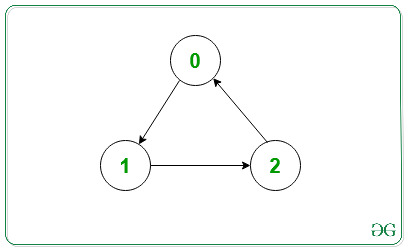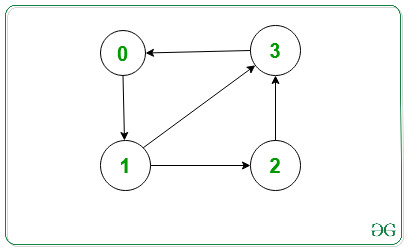# Print Adjacency List for a Directed Graph

• Last Updated : 11 Feb, 2022

An Adjacency List is used for representing graphs. Here, for every vertex in the graph, we have a list of all the other vertices which the particular vertex has an edge to.

Problem: Given the adjacency list and number of vertices and edges of a graph, the task is to represent the adjacency list for a directed graph.

Examples:

Input: V = 3, edges[][]= {{0, 1}, {1, 2} {2, 0}}Output:   0 -> 1
1 -> 2
2 -> 0
Explanation:
The output represents the adjacency list for the given graph.

Input: V = 4, edges[][] = {{0, 1}, {1, 2}, {1, 3}, {2, 3}, {3, 0}}Output:   0 -> 1
1 -> 2 3
2 -> 3
3 -> 0
Explanation:
The output represents the adjacency list for the given graph.

Approach (using STL): The main idea is to represent the graph as an array of vectors such that every vector represents the adjacency list of a single vertex. Using STL, the code becomes simpler and easier to understand.

Below is the implementation of the above approach:

## C++

 `// C++ program for the above approach` `#include ``using` `namespace` `std;` `// Function to add edges``void` `addEdge(vector<``int``> adj[], ``int` `u, ``int` `v)``{``    ``adj[u].push_back(v);``}` `// Function to print adjacency list``void` `adjacencylist(vector<``int``> adj[], ``int` `V)``{``    ``for` `(``int` `i = 0; i < V; i++) {``        ``cout << i << ``"->"``;``        ``for` `(``int``& x : adj[i]) {``            ``cout << x << ``" "``;``        ``}``        ``cout << endl;``    ``}``}` `// Function to initialize the adjacency list``// of the given graph``void` `initGraph(``int` `V, ``int` `edges, ``int` `noOfEdges)``{``    ``// To represent graph as adjacency list``    ``vector<``int``> adj[V];` `    ``// Traverse edges array and make edges``    ``for` `(``int` `i = 0; i < noOfEdges; i++) {` `        ``// Function call to make an edge``        ``addEdge(adj, edges[i], edges[i]);``    ``}` `    ``// Function Call to print adjacency list``    ``adjacencylist(adj, V);``}` `// Driver Code``int` `main()``{``    ``// Given vertices``    ``int` `V = 3;` `    ``// Given edges``    ``int` `edges = { { 0, 1 }, { 1, 2 }, { 2, 0 } };` `    ``int` `noOfEdges = 3;` `    ``// Function Call``    ``initGraph(V, edges, noOfEdges);` `    ``return` `0;``}`

## Java

 `// Java program for the above approach``import` `java.util.*;` `class` `GFG``{` `  ``// Function to add edges``  ``static` `void` `addEdge(Vector adj[], ``int` `u, ``int` `v)``  ``{``    ``adj[u].add(v);``  ``}` `  ``// Function to print adjacency list``  ``static` `void` `adjacencylist(Vector adj[], ``int` `V)``  ``{``    ``for` `(``int` `i = ``0``; i < V; i++) {``      ``System.out.print(i+ ``"->"``);``      ``for` `(``int` `x : adj[i]) {``        ``System.out.print(x+ ``" "``);``      ``}``      ``System.out.println();``    ``}``  ``}` `  ``// Function to initialize the adjacency list``  ``// of the given graph``  ``static` `void` `initGraph(``int` `V, ``int` `edges[][], ``int` `noOfEdges)``  ``{` `    ``// To represent graph as adjacency list``    ``@SuppressWarnings``(``"unchecked"``)``    ``Vector adj[] = ``new` `Vector[``3``];``    ``for``(``int` `i =``0``;i();``    ``}``    ``// Traverse edges array and make edges``    ``for` `(``int` `i = ``0``; i < noOfEdges; i++) {` `      ``// Function call to make an edge``      ``addEdge(adj, edges[i][``0``], edges[i][``1``]);``    ``}` `    ``// Function Call to print adjacency list``    ``adjacencylist(adj, V);``  ``}` `  ``// Driver Code``  ``public` `static` `void` `main(String[] args)``  ``{``    ``// Given vertices``    ``int` `V = ``3``;` `    ``// Given edges``    ``int` `edges[][] = { { ``0``, ``1` `}, { ``1``, ``2` `}, { ``2``, ``0` `} };` `    ``int` `noOfEdges = ``3``;` `    ``// Function Call``    ``initGraph(V, edges, noOfEdges);` `  ``}``}` `// This code is contributed by gauravrajput1`

## Python3

 `# Python program for the above approach` `# Function to add edges``def` `addEdge(adj, u, v):``    ``adj[u].append(v)``  ` `# Function to print adjacency list``def` `adjacencylist(adj, V):``    ` `    ``for` `i ``in` `range` `(``0``, V):``        ``print``(i, ``"->"``, end``=``"")``        ` `        ``for` `x ``in`  `adj[i]:``            ``print``(x , ``" "``, end``=``"")``      ` `        ``print``()``    ` `# Function to initialize the adjacency list``# of the given graph``def` `initGraph(V, edges, noOfEdges):` `    ``adj ``=` `[``0``]``*` `3``    ` `    ``for` `i ``in` `range``(``0``, ``len``(adj)):``        ``adj[i] ``=` `[]``  ` `    ``# Traverse edges array and make edges``    ``for` `i ``in` `range``(``0``, noOfEdges) :` `         ``# Function call to make an edge``        ``addEdge(adj, edges[i][``0``], edges[i][``1``])``    `  `    ``# Function Call to print adjacency list``    ``adjacencylist(adj, V)``  ` `# Driver Code``  ` `# Given vertices``V ``=` `3` `# Given edges``edges ``=`  `[[``0``, ``1` `],  [``1``, ``2` `],  [``2``, ``0` `]]` `noOfEdges ``=` `3``;` `# Function Call``initGraph(V, edges, noOfEdges)` `# This code is contributed by AR_Gaurav`

## C#

 `// C# program for the above approach``using` `System;``using` `System.Collections.Generic;` `public` `class` `GFG``{` `  ``// Function to add edges``  ``static` `void` `addEdge(List<``int``> []adj, ``int` `u, ``int` `v)``  ``{``    ``adj[u].Add(v);``  ``}` `  ``// Function to print adjacency list``  ``static` `void` `adjacencylist(List<``int``> []adj, ``int` `V)``  ``{``    ``for` `(``int` `i = 0; i < V; i++) {``      ``Console.Write(i+ ``"->"``);``      ``foreach` `(``int` `x ``in` `adj[i]) {``        ``Console.Write(x+ ``" "``);``      ``}``      ``Console.WriteLine();``    ``}``  ``}` `  ``// Function to initialize the adjacency list``  ``// of the given graph``  ``static` `void` `initGraph(``int` `V, ``int` `[,]edges, ``int` `noOfEdges)``  ``{` `    ``// To represent graph as adjacency list  ``    ``List<``int``> []adj = ``new` `List<``int``>;``    ``for``(``int` `i = 0; i < adj.Length; i++) {``      ``adj[i] = ``new` `List<``int``>();``    ``}``    ` `    ``// Traverse edges array and make edges``    ``for` `(``int` `i = 0; i < noOfEdges; i++) {` `      ``// Function call to make an edge``      ``addEdge(adj, edges[i,0], edges[i,1]);``    ``}` `    ``// Function Call to print adjacency list``    ``adjacencylist(adj, V);``  ``}` `  ``// Driver Code``  ``public` `static` `void` `Main(String[] args)``  ``{``    ``// Given vertices``    ``int` `V = 3;` `    ``// Given edges``    ``int` `[,]edges = { { 0, 1 }, { 1, 2 }, { 2, 0 } };` `    ``int` `noOfEdges = 3;` `    ``// Function Call``    ``initGraph(V, edges, noOfEdges);` `  ``}``}` `// This code is contributed by Amit Katiyar`

## Javascript

 ``

Output:

```0->1
1->2
2->0```

My Personal Notes arrow_drop_up